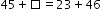# MA.2.AR.2.2Export Print
Determine the unknown whole number in an addition or subtraction equation, relating three or four whole numbers, with the unknown in any position.

### Examples

Determine the unknown in the equation.

### Clarifications

Clarification 1: Instruction extends the development of algebraic thinking skills where the symbolic representation of the unknown uses any symbol other than a letter.

Clarification 2: Problems include having the unknown on either side of the equal sign.

Clarification 3: Addition and subtraction are limited to sums up to 100 and related differences. Refer to Situations Involving Operations with Numbers (Appendix A).

General Information
Subject Area: Mathematics (B.E.S.T.)
Strand: Algebraic Reasoning
Status: State Board Approved

## Related Courses

This benchmark is part of these courses.
5012040: Mathematics - Grade Two (Specifically in versions: 2014 - 2015, 2015 - 2022, 2022 and beyond (current))
7712030: Access Mathematics Grade 2 (Specifically in versions: 2014 - 2015, 2015 - 2018, 2018 - 2022, 2022 and beyond (current))
5012005: Foundational Skills in Mathematics K-2 (Specifically in versions: 2019 - 2022, 2022 and beyond (current))

## Related Access Points

Alternate version of this benchmark for students with significant cognitive disabilities.
MA.2.AR.2.AP.2: Determine the unknown whole number in an addition or subtraction equation, relating three whole numbers, with the change or result unknown (e.g., 7 + _ = 10, 10 − 3= ?). Sums may not exceed 20 and their related subtraction facts.

## Related Resources

Vetted resources educators can use to teach the concepts and skills in this benchmark.

## Formative Assessments

What Number Makes the Equation True?:

Students find the missing number in an equation relating four whole numbers.

Type: Formative Assessment

Relating Four Whole Numbers:

Students find the missing number in an equation relating four whole numbers that involves addition and subtraction.

Type: Formative Assessment

Students find the missing addend in an addition equation relating five whole numbers.

Type: Formative Assessment

## Original Student Tutorial

Balancing Act:

Help Tyler balance equations by finding the unknown number in this interactive tutorial.

Type: Original Student Tutorial

## MFAS Formative Assessments

Students find the missing addend in an addition equation relating five whole numbers.

Relating Four Whole Numbers:

Students find the missing number in an equation relating four whole numbers that involves addition and subtraction.

What Number Makes the Equation True?:

Students find the missing number in an equation relating four whole numbers.

## Original Student Tutorials Mathematics - Grades K-5

Balancing Act:

Help Tyler balance equations by finding the unknown number in this interactive tutorial.

## Student Resources

Vetted resources students can use to learn the concepts and skills in this benchmark.

## Original Student Tutorial

Balancing Act:

Help Tyler balance equations by finding the unknown number in this interactive tutorial.

Type: Original Student Tutorial

## Parent Resources

Vetted resources caregivers can use to help students learn the concepts and skills in this benchmark.